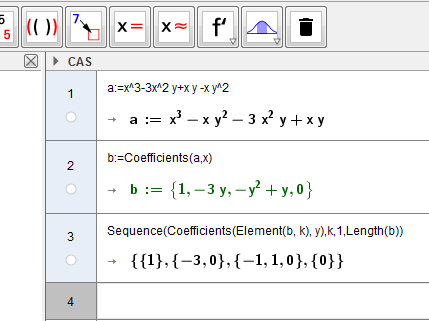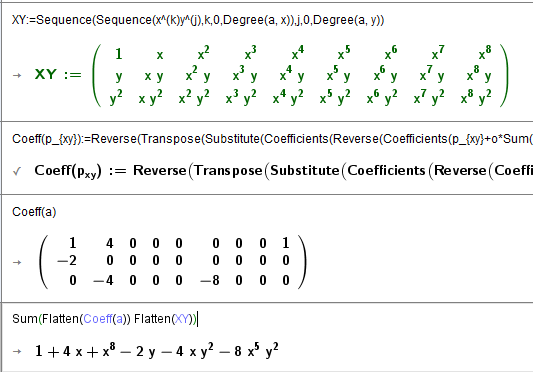# Coefficient matrix of a two variable polynomial

Alicia Cantón shared this question 1 year ago

Hi:

Given a two variable polynomial, I would like to create a matrix with its coefficients so that the M(i,j) element of the matrix should be the coefficient of x^{i-1}*y^{j-1} (or similarly, M(i,j) could be the coefficient of x^(n+1-i)*y(m+1-j) with n the degree in the x variable and m the degree in the y variable). In CAS I have iterated the Coefficient command to get a list of lists, but it does not give a matrix. Is there a simple way to get such a matrix?

I have also tried to get those coefficients by taking partial derivatives and evaluating them at the origin. I have defined a matrix with the partial derivatives, but I do not know whether there is a bug in the evaluation code since, although the matrix of derivatives is correct, its evaluation at the origin is not (maybe I am not doing something right).1

use CAS

fill with 0 the rest of elements1

Hi:

I think that your suggestion is equivalent to writing Coefficients(Coefficients(a(x,y),x),y) in CAS which is what I have tried. Precisely, what I would like to avoid is to have to fill the incomplete rows with 0's. I would like to be able to get the matrix of coefficients for a two variable polynomial that one could introduce using an input box, and I do not see an easy way to fill the 0's in that situation.

By the way, do you know why the evaluation of the derivatives at (0,0) does not work?1

easy enough?

Files: foro.ggb1

l5(i,j,0,0) has no sense

you can to use zip instead because (i,j is not necessary

I think a nested derivative is better like in attached

In attached I do not use CAS so you can do a custom tool

Files: foro.ggb1

Wow. Necesito aprender a usar este comando Zip... Saludos1

Thanks to the input from mathmagic I was able to finish my approach

Perhaps you want to combine all one line? As user-function1